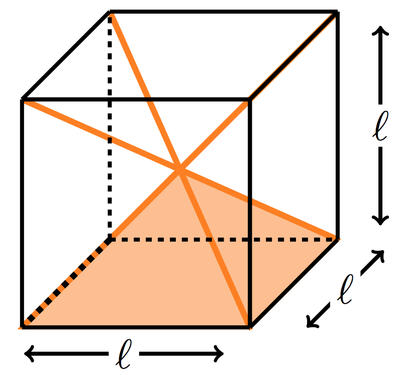# Volume of a Special Pyramid

Alignments to Content Standards: G-GMD.A.1

The four diagonals of a cube with side length $\ell$ meet in a point $P$, and divide the cube into six rectangular pyramids with square bases.

1. What is the height of each of these pyramids?
2. What is the volume of each of these pyramids?
3. It seems reasonable to suppose that the volume of a rectangular pyramid is, like a rectangular prism, proportional to its length $\ell$, width $w$, and height $h$.  That is, we expect a formula of the form $$V=c(\ell wh)$$ for some constant $c$. Find a constant $c$ for which this formula is true for the square pyramid described in the previous parts.
4. Does the disection method described in this problem work to find a formula for the volume of an arbitrary pyramid? Why?

## IM Commentary

The goal of this task is to calculate the volume of a particular pyramid with square base, which is easily reasoned by viewing it as one sixth of a cube.  The method does not apply to other pyramids or to cones but has the advantage of being very concrete. Students might build different shaped pyramids and see what happens when they try to put six of them together. Only when the height of the pyramid is half the length of the sides of the base will they fit together as in this task. It is remarkable that the formula for the volume obtained here, $\frac{1}{3}{\rm area(base)} \times {\rm height}$, applies to all pyramids with square base.

Understanding the formula for the volume of a pyramid is the first step toward the analogous forumla for a cylinder.  Although it is not possible to fit cones together to make a cylinder, the ratio of the volume of a cone to the volume of the cylinder (with equal base and the same height) is the same as the ratio of the square pyramid to the cube (or rectangular prism it is inscribed in). We can see this by taking horizontal slices: for the cone this is circles while for the square pyramid it is squares but in both cases the ratio (area of slice: area of slice of circumscribed shape) is the same for every slice. If we view the three dimensional objects as made up of many very thin slices in this way, this helps explain why the volume of a cone is $\frac{1}{3}$ of the cylinder in which it is inscribed. This idea is called Cavalieri's principle and the basic idea dates back at least to Archimedes.

## Solution

Here is a picture of the scenario.  Note that the congruence of the 6 pyramids can be viewed either dynamically (rotating the cube takes one pyramid onto another) or by calculating that all correpsonding lengths are equal.1. The height of the pyramid is the distance from the peak to its base.  Since the peak of the pyramid is the center of the cube, it halfway between any pair of opposite faces of the cube.  Since opposite faces of the cube are $\ell$ units apart, the height of the pyramid is $\ell/2$.

2. Since the 6 identical pyramids perfectly fill the cube, the volume of each pyramid is $\frac{1}{6}$ of the surrounding cube, or $\frac{1}{6}\ell^3$.

3. For our pyramid, we have $w=\ell$, and $h=\ell/2$, so the formula given in the problem is $V=c(\ell)(\ell)(\ell/2)=\frac{c}{2}\,\ell^3$.  For this to match our volume calculation from the previous part, we need to have $c=\frac{1}{3}$.  That is, we find that for this particular pyramid, we have the formula $$V=\frac{1}{3}(\ell wh).$$

4. The method used here is very special to this particular shape of pyramid. First it is vital that the base of the pyramid is a square. Secondly it is vital that the height of the pyramid be half the length of the base sides so that the six pyramids fit together perfectly to make a cube. If the height were smaller or larger they would no longer fit together to make a cube and so some other argument would be needed to calculate the volume.